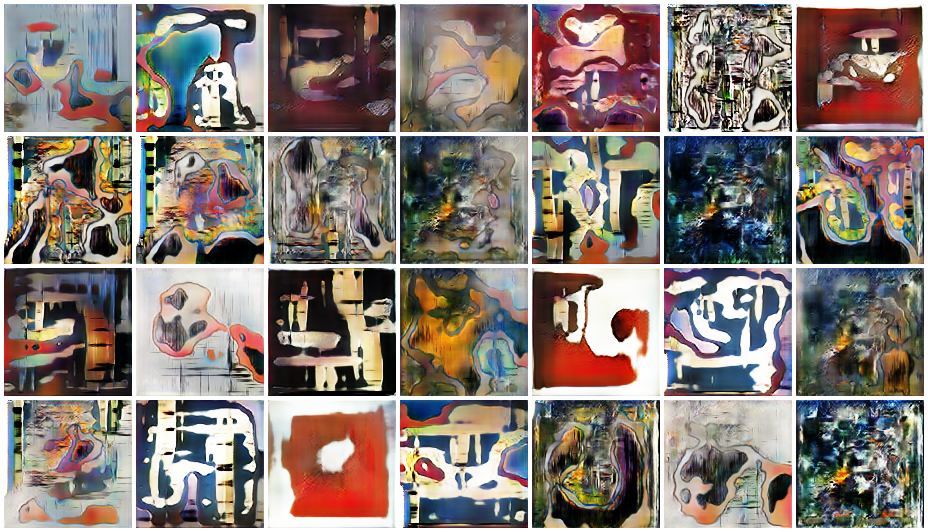# Generating Modern Arts using Generative Adversarial Network(GAN) on Spell

Source: Deep Learning on MediumAfter initializing both generator and discriminator model, let’s write a helper function to save the image after some iteration.

`def save_images(cnt, noise): image_array = np.full(( PREVIEW_MARGIN + (PREVIEW_ROWS * (IMAGE_SIZE + PREVIEW_MARGIN)), PREVIEW_MARGIN + (PREVIEW_COLS * (IMAGE_SIZE + PREVIEW_MARGIN)), 3), 255, dtype=np.uint8)generated_images = generator.predict(noise)generated_images = 0.5 * generated_images + 0.5image_count = 0for row in range(PREVIEW_ROWS):for col in range(PREVIEW_COLS): r = row * (IMAGE_SIZE + PREVIEW_MARGIN) + PREVIEW_MARGIN c = col * (IMAGE_SIZE + PREVIEW_MARGIN) + PREVIEW_MARGIN image_array[r:r + IMAGE_SIZE, c:c + IMAGE_SIZE] = generated_images[image_count] * 255 image_count += 1output_path = 'output' if not os.path.exists(output_path): os.makedirs(output_path)filename = os.path.join(output_path, f"trained-{cnt}.png") im = Image.fromarray(image_array) im.save(filename)`

Our save_images function takes count and a noise as an input.

Inside the function it generates a frames from the parameters we’ve defined above and stores our generated images array which are generated from the noise input.

After that, it saves it as an image.

Now, it’s time for us to compile the models and train them.

Let’s write a block of code for that as well:

`image_shape = (IMAGE_SIZE, IMAGE_SIZE, IMAGE_CHANNELS)optimizer = Adam(1.5e-4, 0.5)discriminator = build_discriminator(image_shape)discriminator.compile(loss=”binary_crossentropy”,optimizer=optimizer, metrics=[“accuracy”])generator = build_generator(NOISE_SIZE, IMAGE_CHANNELS)random_input = Input(shape=(NOISE_SIZE,))generated_image = generator(random_input)discriminator.trainable = Falsevalidity = discriminator(generated_image)combined = Model(random_input, validity)combined.compile(loss=”binary_crossentropy”,optimizer=optimizer, metrics=[“accuracy”])y_real = np.ones((BATCH_SIZE, 1))y_fake = np.zeros((BATCH_SIZE, 1))fixed_noise = np.random.normal(0, 1, (PREVIEW_ROWS * PREVIEW_COLS, NOISE_SIZE))cnt = 1for epoch in range(EPOCHS): idx = np.random.randint(0, training_data.shape, BATCH_SIZE) x_real = training_data[idx] noise= np.random.normal(0, 1, (BATCH_SIZE, NOISE_SIZE)) x_fake = generator.predict(noise) discriminator_metric_real = discriminator.train_on_batch(x_real, y_real)discriminator_metric_generated = discriminator.train_on_batch( x_fake, y_fake)discriminator_metric = 0.5 * np.add(discriminator_metric_real, discriminator_metric_generated)generator_metric = combined.train_on_batch(noise, y_real)if epoch % SAVE_FREQ == 0: save_images(cnt, fixed_noise) cnt += 1 print(f”{epoch} epoch, Discriminator accuracy: {100* discriminator_metric}, Generator accuracy: {100 * generator_metric}”)`

Breaking it down:

Here in the first few lines, we have defined our input shape: which is 128X128X3 (image_size, image_size, image_channel).

After that we are using Adam as our optimizer.

Note: All the parameters has been sourced from the paper .

`image_shape = (IMAGE_SIZE, IMAGE_SIZE, IMAGE_CHANNELS)optimizer = Adam(1.5e-4, 0.5)discriminator = build_discriminator(image_shape)discriminator.compile(loss=”binary_crossentropy”,optimizer=optimizer, metrics=[“accuracy”])`

After initializing the optimizer, we are calling our buid_discriminator function and passing the image shape then compiling it with a loss function and an optimizer.

Since it is a classification model, we are using accuracy as it’s performance metric.

Similarly, in the next line we are calling our build_generator function and passing our random_input noise vector as it’s input.

`generator = build_generator(NOISE_SIZE, IMAGE_CHANNELS)random_input = Input(shape=(NOISE_SIZE,))generated_image = generator(random_input)`

It returns a generated image as it’s output.

Now, one important part for GAN is we should prevent our discriminator from training.

`discriminator.trainable = Falsevalidity = discriminator(generated_image)combined = Model(random_input, validity)combined.compile(loss=”binary_crossentropy”,optimizer=optimizer, metrics=[“accuracy”])`

Since we are only training generators here, we do not want to adjust the weights of discriminator.

If we do not set this, the generator will get its weight adjusted so it gets better at fooling discriminator and it also adjust the weights of discriminator to make it better at being fooled.

We don’t want this. So, we have to train them separately and fight against each other.

We are then compiling the generative model with loss function and optimizer.

After that we are defining two vectors as y_real and y_fake.

`y_real = np.ones((BATCH_SIZE, 1))y_fake = np.zeros((BATCH_SIZE, 1))fixed_noise = np.random.normal(0, 1, (PREVIEW_ROWS * PREVIEW_COLS, NOISE_SIZE))`

These vectors are composed of randomly random 0’s and 1’s values.

After that we are creating a fixed_noise: this will result in generating images which are saved later on which we can see being getting better on every iteration.

After that, we are going to iterate over our training data with the range of epochs we’ve defined.

`cnt = 1for epoch in range(EPOCHS): idx = np.random.randint(0, training_data.shape, BATCH_SIZE) x_real = training_data[idx] noise= np.random.normal(0, 1, (BATCH_SIZE, NOISE_SIZE)) x_fake = generator.predict(noise) discriminator_metric_real = discriminator.train_on_batch(x_real, y_real) discriminator_metric_generated = discriminator.train_on_batch( x_fake, y_fake) discriminator_metric = 0.5 * np.add(discriminator_metric_real, discriminator_metric_generated) generator_metric = combined.train_on_batch(noisse, y_real)if epoch % SAVE_FREQ == 0: save_images(cnt, fixed_noise) cnt += 1 print(f”{epoch} epoch, Discriminator accuracy: {100* discriminator_metric}, Generator accuracy: {100 * generator_metric}”)`

During the iteration process, we are taking a sample from real image and putting that on x_real. After that we are defining a noise vector and passing that to our generator model to generate fake image in x_fake.

Then we are training our discriminator model in both real and fake images separately.

`discriminator_metric_real = discriminator.train_on_batch(x_real, y_real)discriminator_metric_generated = discriminator.train_on_batch( x_fake, y_fake)`

Some research has shown that training them separately has can get us some better results.

After training, we are taking the metric from both model and taking the average.

`discriminator_metric = 0.5 * np.add(discriminator_metric_real, discriminator_metric_generated)`

This way we get the metric for discriminator model, now for generator model, we are training it on our noise vector and y_real: which is a vector of 1’s.

Here we are trying to train the generator. Overtime generator will get better from these inputs and discriminator will not be able to discriminate whether the input is fake or real.

One thing to note here, our combined model is based on the generator model linked directly to the discriminator model. Here our Input is what generator wants as an input: which is a noise and output is what discriminator gives us.

Now in the end we have an if statement which checks for our checkpoint.

`if epoch % SAVE_FREQ == 0: save_images(cnt, fixed_noise) cnt += 1 print(f”{epoch} epoch, Discriminator accuracy: {100* discriminator_metric}, Generator accuracy: {100 * generator_metric}”)`

If it reaches the checkpoint then it saves the current iteration noise and prints the current accuracy of generator and discriminator.

This is all for the coding part to create GAN, but we are not finished yet.

We have just written a code for it, now we have to actually train those models and see the output how it performs.